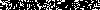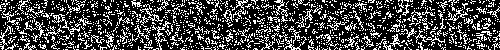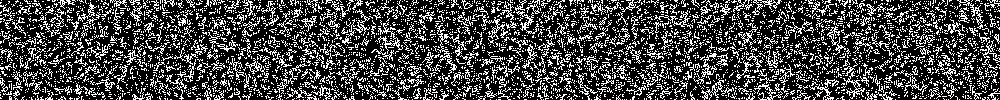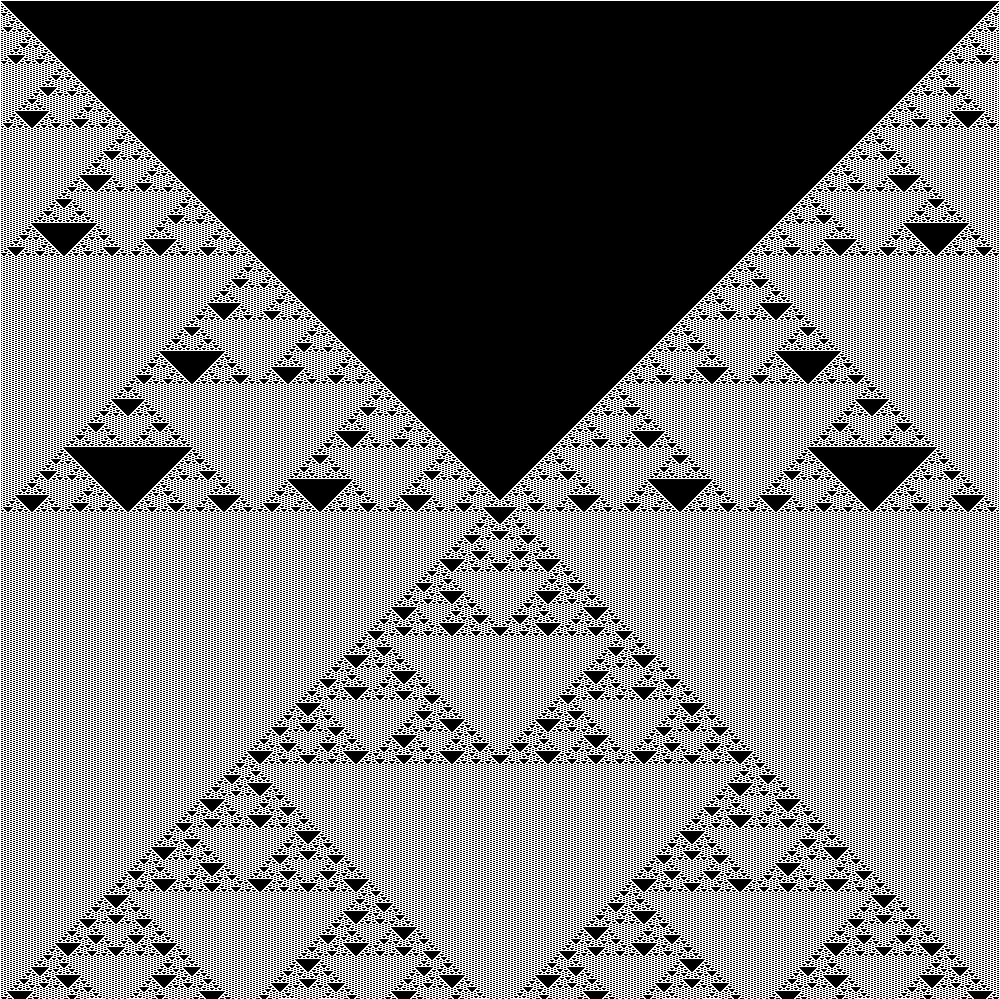# Conway's Life## A Python Programming Primer### Conway's Life: One Dimensional Bitwise Operators

```import numpy as np
from skimage.io import imsave

def game_life_image(life, width, length, sN):
'''creates image showing linear evolution of Conways Game of Life
seed (life) value at top, evolution proceedes linearly downward

life = seed value, initial state, any integer value under 2**width
width = size of image (width of playing field)
length = height of image (number of turns)
sN = save name for image file
'''

img = []
for x in range(1,length):

print '{: <4}  {}'.format(x, np.binary_repr(life,width))
img.append([float(i) for i in np.binary_repr(life,width)])

#evolution logic using bitwise operators
life = (life | life<<1 | life>>1) & ~(life & life<<1 & life>>1)

#eliminates overflow, keeping image at a constant width
if life > 2**width:
life -= 2**width

img = np.array(img)
print img.shape
print img
imsave(sN, img)

def one_dimensional_game_life_samples():
'''creates a series of sample images for game_life_image()

manually change w and testValues as desired
'''
w = 250
testValues = [(0,w,w, "life_%d_%d_0.png" % (w,w)),
(1,w,w, "life_%d_%d_1.png" % (w,w)),
(471,w,w*10, "life_%d_%d_471.png" % (w,w*10)),
(int('1110000000'*(w/10),2),w,w*10,
"life_%d_%d_1110000000.png" % (w,w*10)),
(int('10101'*(w/5),2),w,w*10,
"life_%d_%d_10101.png" % (w,w*10)),
(int('11100'*(w/5),2),w,w*10,
"life_%d_%d_11100.png" % (w,w*10)),
(int('0111001110'*(w/10),2),w,w*10,
"life_%d_%d_0111001110.png" % (w,w*10)),
]

for a,b,c,d in testValues:
game_life_image(a,b,c,d)

one_dimensional_game_life_samples()
```

The Last Four Images Thus Derived

### Conway's Life: Two Dimensional using Numpy Roll

```import numpy as np
import moviepy.editor as mpy

def evolution(seed):
'''Conway's life two-dimensional turn logic

given seed, returns next incremental step in Game of Life
'''

#Cheesy, perhaps, but it gets the job done
#These shift the array, up, down, right, left, etc.
down = np.roll(seed,1,0)
up = np.roll(seed,-1,0)
right = np.roll(seed,1,1)
left = np.roll(seed,-1,1)
upRight = np.roll(right,-1,0)
upLeft = np.roll(left,-1,0)
downRight = np.roll(right,1,0)
downLeft = np.roll(left,1,0)

#adding all the shifted arrays together, overweighting original
s = (down + up + right + left +
upRight + upLeft + downRight + downLeft + 10*seed)

#logic of the Game of Life
s[s==1.0] = 0.0
s[s==13.0] = 1.0
s[s==12.0] = 1.0
s[s==3.0] = 1.0
s[s!=1.0] = 0.0
print s

return s

def two_dimensional_life_samples():
'''convenience function to quickly create series of gifs

manually change testValues as appropriate
'''

testValues = [(np.array(np.random.randint(0,2,(50,500)), float),
250,
'conway_life_250frames_50x500.gif'),
(np.array(np.random.randint(0,2,(10,100)), float),
250,
'conway_life_250frames_10x100.gif'),
(np.array(np.random.randint(0,2,(100,1000)), float),
250,
'conway_life_250frames_100x1000.gif'),
]
for seed,frames,sN in testValues:

#holding array for stacked image-like arrays
gifArray = []

for _ in range(frames):
seed = evolution(seed)
gifArray.append(seed*255) #255 converts 0.0-1.0 to 0-255

#using the moviepy library to make a gif
clip = mpy.ImageSequenceClip(gifArray, fps=5)
clip.write_gif(sN)

two_dimensional_life_samples()
```

With the Images Thus Derived Closing Out the PageFor More Code
Brett CodeFor Only the Greatest Story Ever Written
Minataur Tailswww.Paufler.netConway's Life: 1000x1000 All Ones Seed

paufler.net@gmail.com